# Stacks and Queues What are stacks and queues

• Slides: 17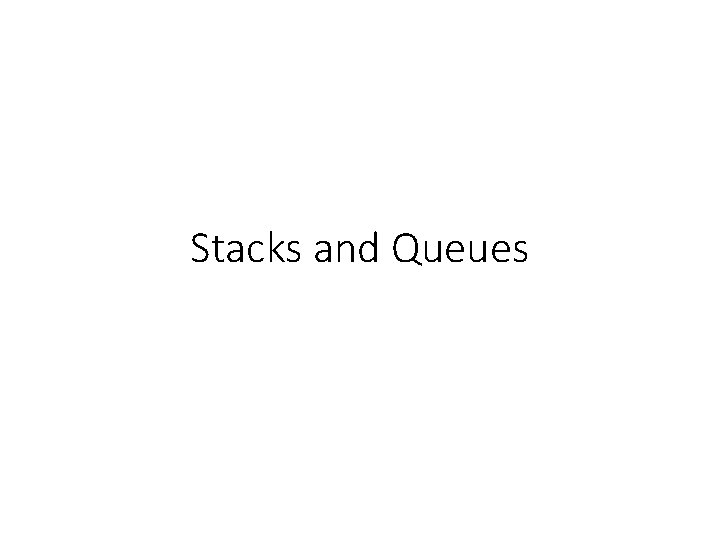Stacks and Queues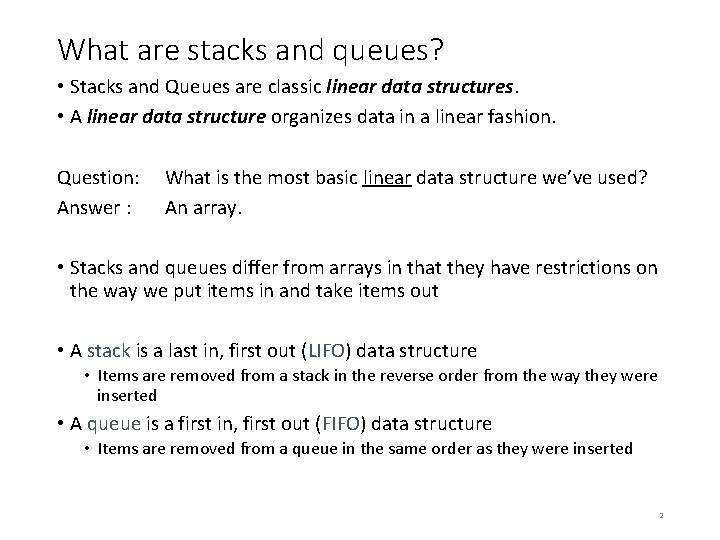What are stacks and queues? • Stacks and Queues are classic linear data structures. • A linear data structure organizes data in a linear fashion. Question: Answer : What is the most basic linear data structure we’ve used? An array. • Stacks and queues differ from arrays in that they have restrictions on the way we put items in and take items out • A stack is a last in, first out (LIFO) data structure • Items are removed from a stack in the reverse order from the way they were inserted • A queue is a first in, first out (FIFO) data structure • Items are removed from a queue in the same order as they were inserted 2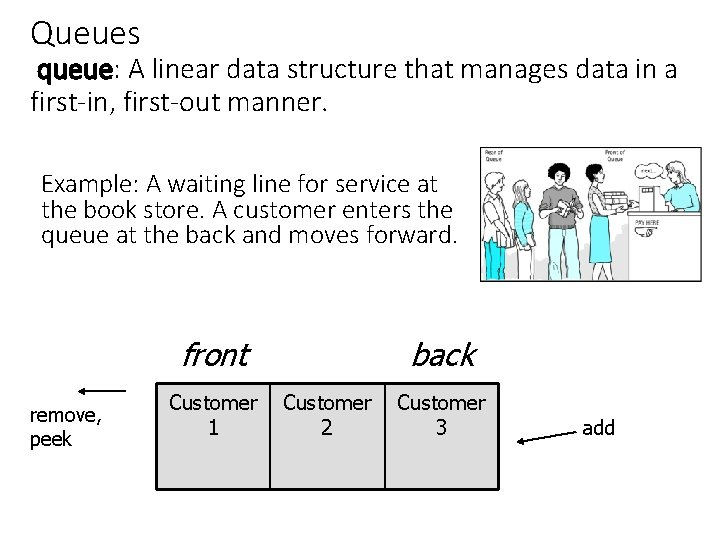Queues queue: A linear data structure that manages data in a first-in, first-out manner. Example: A waiting line for service at the book store. A customer enters the queue at the back and moves forward. front remove, peek Customer 1 back Customer 2 Customer 3 add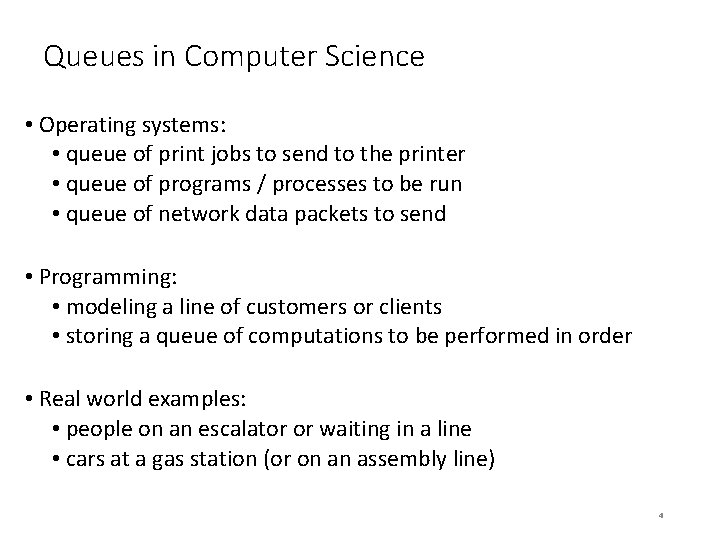Queues in Computer Science • Operating systems: • queue of print jobs to send to the printer • queue of programs / processes to be run • queue of network data packets to send • Programming: • modeling a line of customers or clients • storing a queue of computations to be performed in order • Real world examples: • people on an escalator or waiting in a line • cars at a gas station (or on an assembly line) 4Basic Queue Operations add(obj) places a given object at the back of queue remove() removes an object from front of queue and returns it; throws a No. Such. Element. Exception if queue is empty peek() returns front object from queue without removing it; returns null if queue is empty size() returns the number of objects in queue is. Empty() returns true if queue has no objectsLinked-list implementation of queues • In a queue, insertions occur at the back of the list. • Deletions occur at the front of the list. • These operations can be easily implemented using a singly-linked list (SLL). • You always need a pointer to the head of the list • You can keep an additional pointer to the last item in the list. my. Queue: last head 44 97 23 17 6Adding a node to the Queue Node to add last first 44 97 23 17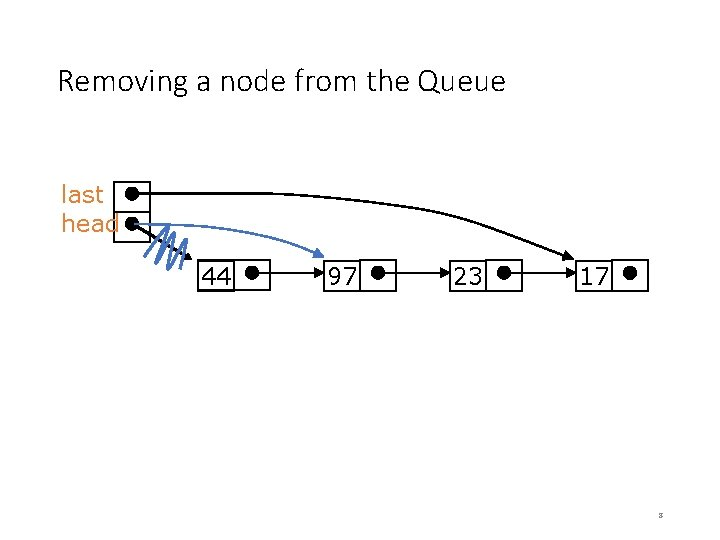Removing a node from the Queue last head 44 97 23 17 8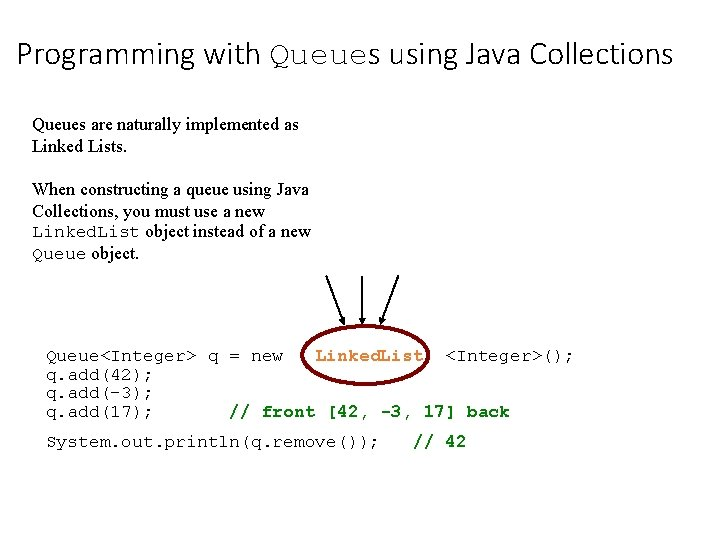Programming with Queues using Java Collections Queues are naturally implemented as Linked Lists. When constructing a queue using Java Collections, you must use a new Linked. List object instead of a new Queue object. Queue<Integer> q = new Linked. List <Integer>(); q. add(42); q. add(-3); q. add(17); // front [42, -3, 17] back System. out. println(q. remove()); // 42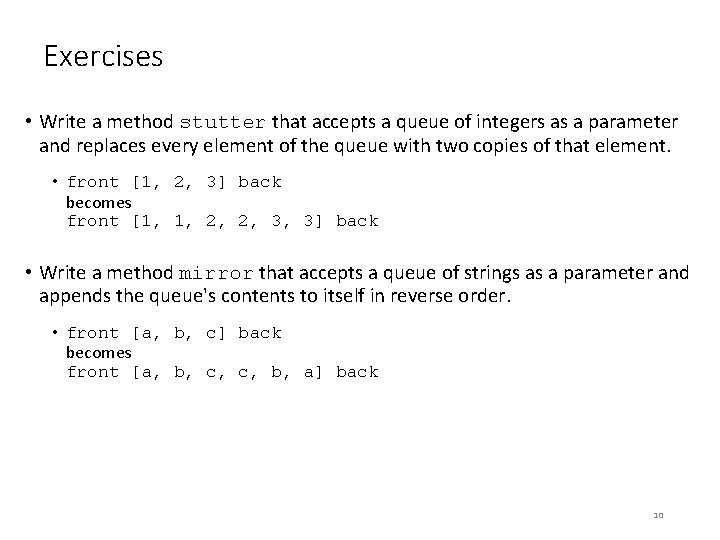Exercises • Write a method stutter that accepts a queue of integers as a parameter and replaces every element of the queue with two copies of that element. • front [1, 2, 3] back becomes front [1, 1, 2, 2, 3, 3] back • Write a method mirror that accepts a queue of strings as a parameter and appends the queue's contents to itself in reverse order. • front [a, b, c] back becomes front [a, b, c, c, b, a] back 10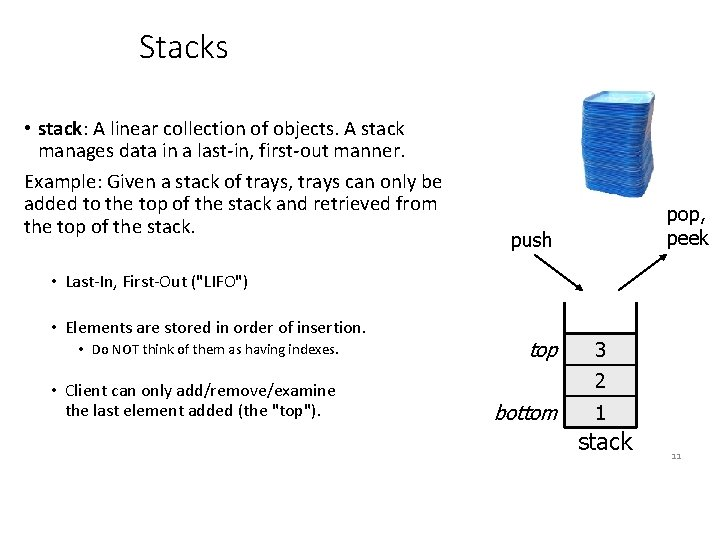Stacks • stack: A linear collection of objects. A stack manages data in a last-in, first-out manner. Example: Given a stack of trays, trays can only be added to the top of the stack and retrieved from the top of the stack. pop, peek push • Last-In, First-Out ("LIFO") • Elements are stored in order of insertion. • Do NOT think of them as having indexes. • Client can only add/remove/examine the last element added (the "top"). top 3 2 bottom 1 stack 11Linked-list implementation of stacks • A singly-linked list (SLL) is an obvious method for implementing a stack. • A top pointer of the list will point to the top (back) of the stack. • pushing is inserting an element at the top of the list. • Popping is removing an element from the top of the list • Actions may only happen at the top of a stack. my. Stack top head 44 97 23 17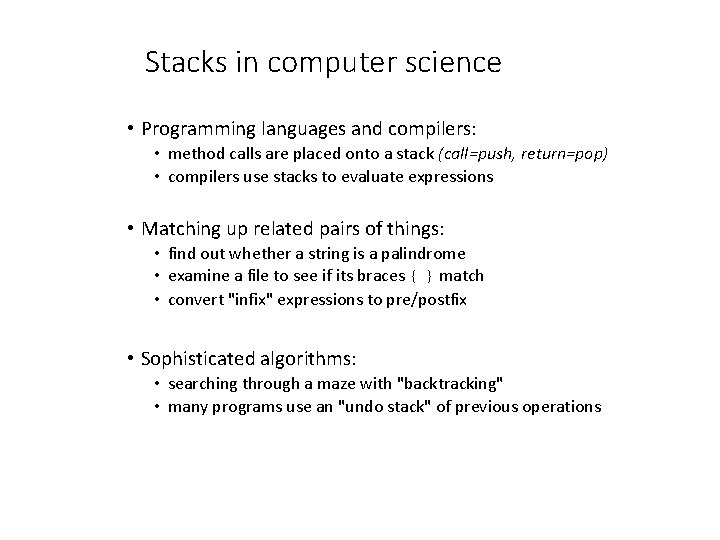Stacks in computer science • Programming languages and compilers: • method calls are placed onto a stack (call=push, return=pop) • compilers use stacks to evaluate expressions • Matching up related pairs of things: • find out whether a string is a palindrome • examine a file to see if its braces { } match • convert "infix" expressions to pre/postfix • Sophisticated algorithms: • searching through a maze with "backtracking" • many programs use an "undo stack" of previous operations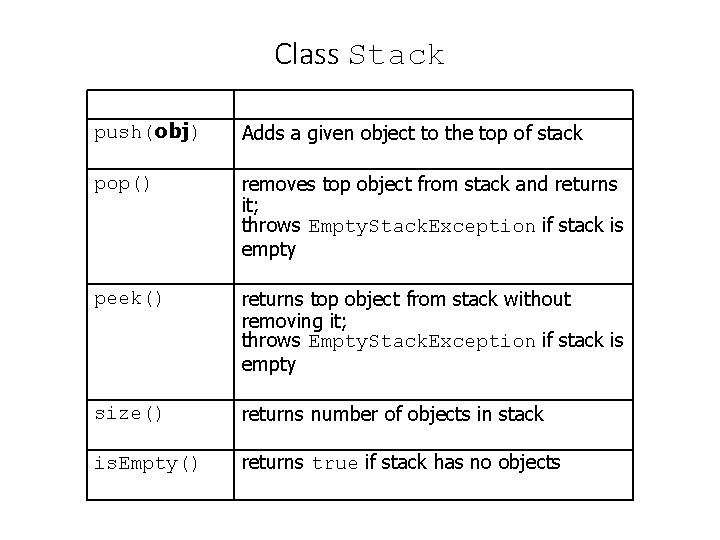Class Stack push(obj) Adds a given object to the top of stack pop() removes top object from stack and returns it; throws Empty. Stack. Exception if stack is empty peek() returns top object from stack without removing it; throws Empty. Stack. Exception if stack is empty size() returns number of objects in stack is. Empty() returns true if stack has no objects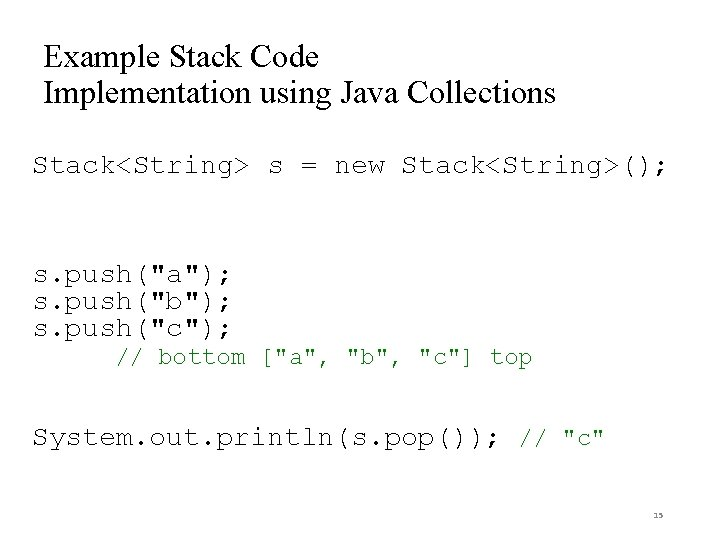Example Stack Code Implementation using Java Collections Stack<String> s = new Stack<String>(); s. push("a"); s. push("b"); s. push("c"); // bottom ["a", "b", "c"] top System. out. println(s. pop()); // "c" 15What is the following stack Logic Error? Problem: Suppose we're asked to write a method max that accepts a Stack of integers and returns the largest integer in the stack. // Assume the stack is not empty. public static void max(Stack<Integer> s) { int max. Value = s. pop(); } while (!s. is. Empty()) { int next = s. pop(); max. Value = Math. max(max. Value, next); } return max. Value; • The algorithm is correct, but what is wrong with the code? 16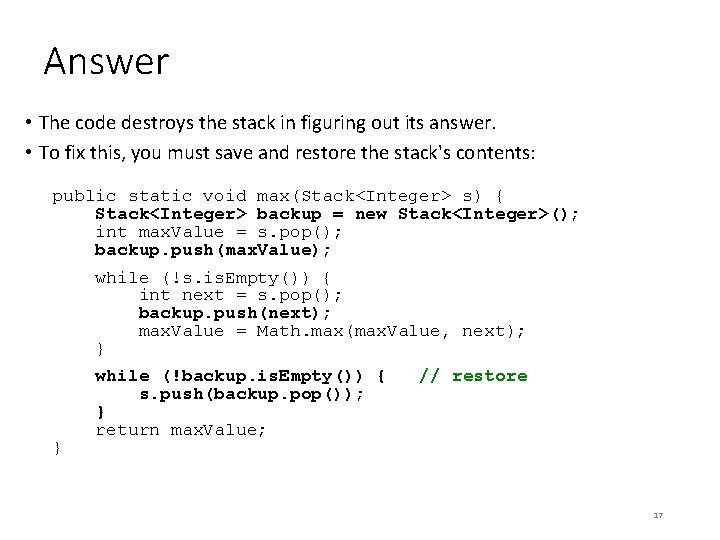Answer • The code destroys the stack in figuring out its answer. • To fix this, you must save and restore the stack's contents: public static void max(Stack<Integer> s) { Stack<Integer> backup = new Stack<Integer>(); int max. Value = s. pop(); backup. push(max. Value); while (!s. is. Empty()) { int next = s. pop(); backup. push(next); max. Value = Math. max(max. Value, next); } } while (!backup. is. Empty()) { s. push(backup. pop()); } return max. Value; // restore 17